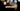# Leetcode | Solution of Guess Number Higher or Lower in JavaScript

May 11th, 2020
|

In this post, we will solve Guess Number Higher or Lower from leetcode and compute it's time and space complexities. Let's begin.

# Problem Statement

The question can be found at leetcode Guess Number Higher or Lower problem.

The problem states that we need to guess the correct number between `1 to n` which the problem has picked based on the following rules

# Constraints and challenges

We are given a guess API which returns

• `1` if the picked number is higher than our guessed number
• `0` if it is equal to the guessed number
• `-1` if the guessed number is lower than the picked number

# Solution

The problem is nothing but a binary search. In the series, `1...n` find a given number. I wish I had more to explain here, but it is a simple binary search. If you don't recall the algorithm, please read about it and then you won't need to read furtherHere is the solution is you are still reading this.

``````var guessNumber = function (n) {

if (guess(n) === 0) return n

let low = 1, high = n;
while (n > 0) {
const pick = (parseInt(high + low) / 2);
const res = guess(pick);

if (res === 0) {
return pick
}
if (res === -1) {
high = pick;
}
if (res === 1) {
low = pick;
}
}
};``````

First, take care of an edge case. If `n` is the picked number we return it first thing in the program. Next, we apply a binary search to find the number.

Here are the stats on submission

``````Status: Accepted
Runtime: 48ms
Memory: 33.7MB``````

## Time and space complexity

### Time complexity

The time complexity here is logarithmic because the input size decreases by half every time. O(log n).

### Space complexity

We are not using any extra space except for a couple of variables. So space complexity is constant, O(1).

# Summary

So, we solved the Guess Number Higher or Lower problem by using binar search and calculated the time and space complexities.

I hope you enjoyed solving this question. This is it for this one, complete source code for this post can be found on my Github Repo. Will see you in the next one.